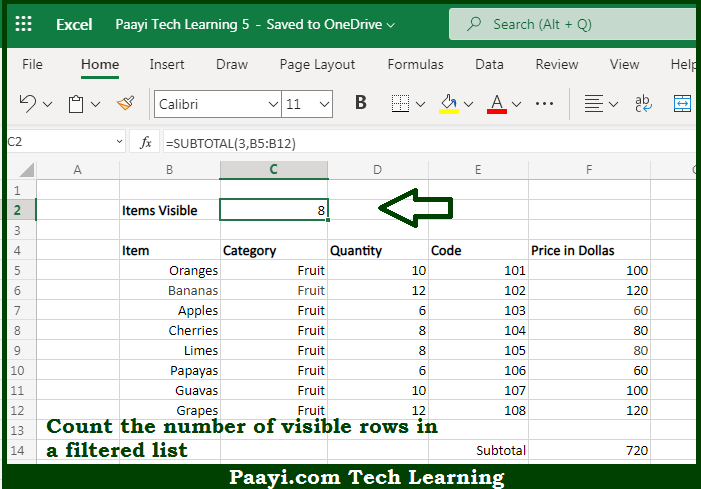# Learn How to COUNT Visible Rows in a Filtered List in Microsoft Excel

Written by | 0 Comments | 595 Views

In this article, you will learn how to COUNT various things in Microsoft Excel using a single or combination of functions and its purpose. You will also get to know how to COUNT Visible Rows in a Filtered List and see the generic formula.

COUNT Visible Rows in a Filtered List in Microsoft Excel

The main purpose of this formula is to count the number of visible rows in a filtered list. Here we will learn how to count visible rows in a filtered list in Microsoft Excel. That implies, with the help of a formula based on the SUBTOTAL function you can able to count the number of visible rows in a filtered list. So, with the help of this formula, you can able to count the number of visible rows in a filtered list.

General Formula to COUNT Visible Rows in a Filtered List

=SUBTOTAL(3,range)

The Explanation for the COUNT Visible Rows in a Filtered ListSo we know that with the help of the given formula above you can able to count the number of visible rows in a filtered list. Here we will learn how to count visible rows in a filtered list in Microsoft Excel. As we know that the SUBTOTAL function is used for calculations like COUNT, SUM, MAX, MIN, and much more. The quality that makes the SUBTOTAL function especially interesting and useful is that it automatically ignores items that are not visible in a filtered list or table. This makes it ideal for showing how many items are visible in a list, the subtotal of visible rows, etc. So, with the help of this formula, you can able to count the number of visible rows in a filtered list.